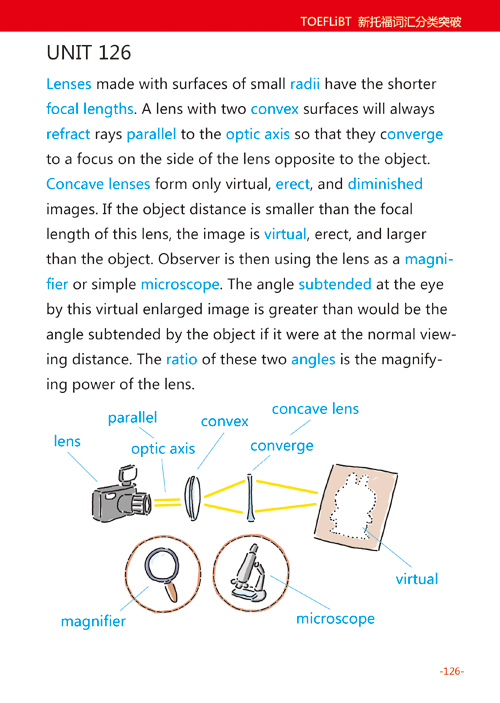# 新托福iBT词汇分类突破 – Unit 126

by on 2009/12/08

Lenses made with surfaces of small radius have the shorter focal lengths. A lens with two convex surfaces will always refract rays parallel to the optic axis so that they converge to a focus on the side of the lens opposite to the object. Concave lenses form only virtual, erect, and diminished images. If the object distance is smaller than the focal length of this lens, the image is virtual, erect, and larger than the object. Observer is then using the lens as a magnifier or simple microscope. The angle subtended at the eye by this virtual enlarged image is greater than would be the angle subtended by the object if it were at the normal viewing distance. The ratio of these two angles is the magnifying power of the lens.

• lens [lenz] n.镜头
• focal length [ˈfəukəl - leŋθ] n.焦距
• refract [riˈfrækt]v.使折射